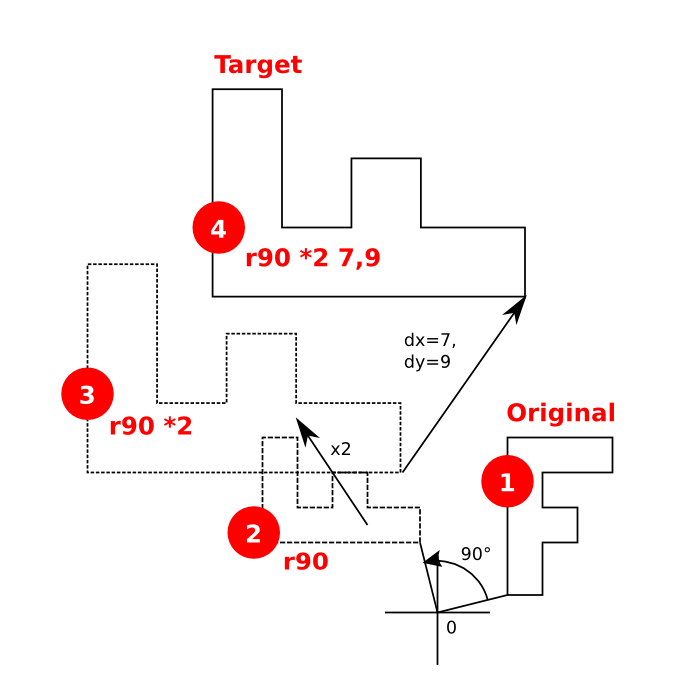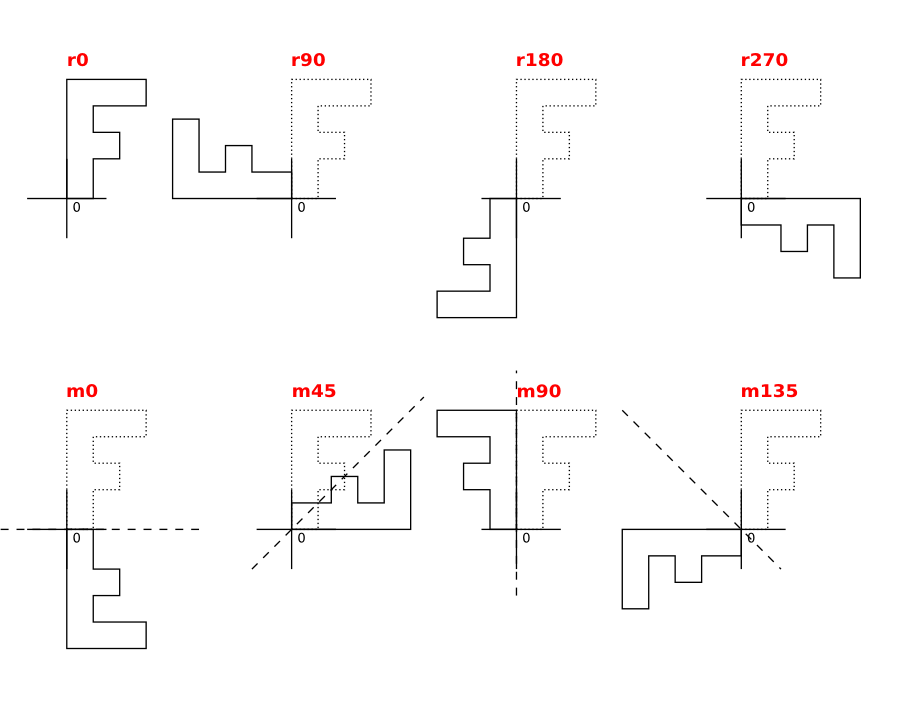Transformations in KLayout

KLayout supports a subset of affine transformations with the following contributions:

• Rotation and/or mirroring: rotation by a given angle or mirroring at a given axis.
• Scaling: magnification by the given factor.
• Translation: a displacement by the given vector.

The execution order is "displacement after rotation, mirroring and scaling". Transformations are used for example to describe the instantiation of a cell. The content of a cell appears in the parent cell after the given transformation has been applied to the content of the cell.

The transformations supported by KLayout cover the transformations employed within GDS2, OASIS and other layout formats. KLayout does not support shearing currently.

The following figure illustrates the effect of the transformation "r90 *2 7,9". This notation specifies a transformation composed of a rotation by 90 degree, a scaling with factor 2 and a displacement by 7 units in x- and 9 units in y-direction. In that example, the "F" shape is first rotated by 90 degree around the origin. Because the "F" is already displaced from the origin, this will also move the "F" shape. The shape then is scaled. Again it will move because every point of the polygon moves away from the origin. Finally it is displaced by the given displacement vector.The notation shown here is used in many places within KLayout. It is basically composed of the following parts which are combined putting one or more blanks in between. The order the parts are specified is arbitrary: the displacement is always applied after the rotation.

• <x>,<y>: A displacement (applied after rotation and scaling) in micron units. If no displacement is specified, "0,0" is assumed.
• r<a> or m<a>: A rotation by angle "a" (in degrees) or mirroring at the "a" axis (the x axis rotated by "a" degree). If no rotation or mirroring is specified, no rotation is assumed.
• *<s>: A scaling by the factor "s". If no scaling is specified, no scaling is assumed.

Here are some examples:

• 0,100: shift 100 units up.
• r90: rotation by 90 degree counterclockwise (positive in the mathematical sense).
• m0: mirroring at the x-axis.
• m45 100,-200: swap x and y (mirror at 45 degree axis), shift 100 units to the right and 200 units down.
• r22.5 *1.25: rotate by 22.5 degree and scale by factor 1.25.

The distance units are usually micron. In some cases (i.e. transformations inside a database), the unit is database units and dx and dy are integer values.

Mirroring and rotation are exclusive and mirroring includes a rotation. In fact, a mirror operation at a certain axis is identical to a mirror operation at the x-axis, followed by a rotation by twice the angle "a". The following figure illustrates rotation and mirroring with the eight basic transformations involving rotations by multiples of 90 degree:KLayout is not restricted to these basic operations. Arbitrary angles are supported (i.e. "r45" or "m22.5"). Usually however, this implies grid snapping and other issues. This also is true for arbitrary scaling values. KLayout is also more effective when using simple transformations involving only rotations by multiples of 90 degree and do not use scaling.